• 一、Opt（最佳置换算法）：（不排序）       写法：上老下新（下面的是最新访问的）       思想：往后看，替换那个未来不使用或者未来最长时间内不被...
一、Opt（最佳置换算法）：（不排序）
写法：上老下新（下面的是最新访问的）
思想：往后看，替换那个未来不使用或者未来最长时间内不被使用的那个，注意看清题目有几个物理块
二、FIFO（先来先服务）：（把最上面的替换，新来的排到最下面）
注意： 如果已经存在了，当再次被访问时，不用移位，这是与LRU的区别！看清题目有几个物理块
三、LRU（最近最久未使用）：（已经存在的页被访问时，要把该页移到最下面，表示最近访问到了）
注意：看清题目有几个物理块
思想：其他的跟FIFO一样，都是把最上面的替换掉，新来的放到最下面。
四、LFU（最少使用置换算法）：（采用计数器）
注意： 计数器 2 位（bit），表示为 x0~3, 预先存进去的访问次数是 0！ 例如： 采用2位计数器，x3 之后，再访问 x，那么就成了 x0 .  还有就是要看清题目是几个物理块
思想：把访问次数最少的替换了，新来的访问次数是 1 。
五、Clock：（有指针）
注意：开始的时候他会告诉你 每个页面的状态（是 0 还是 1 ），如果是 1 ，下面 画一道横线，是 0 的话就不画。  也会告诉你起始指针的位置，
思想： 如果没有目标页面，从指针的位置开始看，如果是 0 ，也就是下面没有下划线，那么替换这个页面，把新进来的页面下面画下划线，且指针移到下一位。 如果指针位置有下划线，那么把下划线去掉且指针下移，重复此步骤，直到遇到没有下划线的页面，把这个页面替换，并且画上下划线，指针下移。 如果5个物理块，但预存了4个，当指针指到空物理块时，把页面放进来，画上下划线，指针下移。


展开全文• 操作系统页面置换算法模拟实现，fifo、lfu、lru、opt，界面由MFC实现
• 采用C/C++编程模拟实现：FIFO算法、LRU算法、LFU算法、NRU算法四个页面置换算法。并设置自动数据生成程序，比较四个算法的缺页出现概率。 四、程序代码： /* FIFO: 简单的先进先出，用队列模拟即可 prio 表示入队...
操作系统实验报告
一、实验名称 ：页面置换算法
二、实验目的：
在实验过程中应用操作系统的理论知识。
三、实验内容：
采用C/C++编程模拟实现：FIFO算法、LRU算法、LFU算法、NRU算法四个页面置换算法。并设置自动数据生成程序，比较四个算法的缺页出现概率。
四、程序代码：
/*
FIFO: 简单的先进先出，用队列模拟即可 prio 表示入队时间（小值先出）
LRU: 淘汰最久没有被访问的页面 prio 表示上一次入队的时间（小值先出）
LFU: 淘汰最少访问次数的页面 prio 表示被访问的次数
NRU: 四类 prio表示状态类数
第0类：没有被访问，没有被修改。00
第1类：没有被访问，已被修改。  01
第2类：已被访问，没有被修改。  10
第3类：已被访问，已被修改     11
*/

#include<bits/stdc++.h>
#define xcx(x) printf("ojbk %d\n",x)
using namespace std ;
const int PAGE_NUM = 20 ;
const int MEM_SIZE = 3 ;
const double NRU_LIMIT = 3 ;
bool in_mem[ PAGE_NUM+1 ] ;
struct page{
int val, prio, pos ; // val:页数 pos:占内存位置
page () {}
page ( int val , int prio , int pos ) {
this->val = val ;
this->prio = prio ;
this->pos = pos ;
}
};

bool operator < ( const page &l , const page &r ) {
if (  l.prio== r.prio ) {
return l.pos < r.pos ;
}
return l.prio > r.prio ;
}
vector< int > CreatSeq ( int n ) { // 随机生成长度为 n 的访问序列
vector< int > ret ;
for( int i=0; i<n; i++ ) {
ret.push_back( rand()%PAGE_NUM+1 ) ;
}
return ret ;
}
void Init ( vector< vector<int> > &ret , vector< bool > &is_miss , const vector< int > &seq ) {
vector< int > e( MEM_SIZE ) ;
memset( in_mem, false, sizeof(in_mem) ) ;
is_miss.clear() ;   ret.clear() ;
for( int i=0; i<seq.size(); i++ ) {
is_miss.push_back( 0 ) ;
ret.push_back( e ) ;
}
}
vector< vector<int> > FIFO ( const vector< int > &seq , vector< bool > &is_miss ) {
vector< vector<int> > ret ;
Init( ret , is_miss , seq ) ;
queue< page > q ;
bool is_full = false ;
int num = 0, mem_pos[ MEM_SIZE ] = {0} ;
for( int i=0; i<seq.size(); i++ ) {
if ( in_mem[seq[i]] == false ) { // 不在mem
is_miss[i] = true ;
if ( is_full == true ) { // mem已满，淘汰
page temp = q.front() ;
q.pop() ;   in_mem[ temp.val ] = false ;
q.push( page( seq[i], i+1, temp.pos ) );    in_mem[ seq[i] ] = true ;
mem_pos[temp.pos] = seq[i] ;
}
else { // mem未满，添加
q.push( page( seq[i], i+1, num ) );
in_mem[ seq[i] ] = true ;
mem_pos[ num++ ] = seq[i] ;
if ( num >= MEM_SIZE ) is_full = true ;
}
}
///存储当前状态
for( int j=0; j<MEM_SIZE; j++ ) {
ret[i][j] = mem_pos[j] ;
}
}
return ret;
}
vector< vector<int> > LRU ( const vector< int > &seq , vector< bool > &is_miss ) {
vector< vector<int> > ret ;
Init( ret , is_miss , seq ) ;
vector< page > q ;
bool is_full = false ;
int num = 0, mem_pos[ MEM_SIZE ] = {0} ;
for( int i=0; i<seq.size(); i++ ) {
if ( in_mem[seq[i]] == false ) { // 不在 mem
is_miss[i] = true ;
if ( is_full == true ) { // mem已满，淘汰
page temp = q ;
q = page( seq[i], i+1, temp.pos ) ;
in_mem[ temp.val ] = false ;    in_mem[ seq[i] ] = true ;
mem_pos[temp.pos] = seq[i] ;
}
else { // mem未满，添加
q.push_back( page( seq[i], i+1, num ) );
in_mem[ seq[i] ] = true ;
mem_pos[ num++ ] = seq[i] ;
if ( num >= MEM_SIZE ) is_full = true ;
}
}
else { // 在 mem
for( int i=0; i<q.size(); i++ ) {
if ( q[i].val == seq[i] ) {
q[i].prio = i ;
}
}
}
sort( q.begin() , q.end() ) ;
///存储当前状态
for( int j=0; j<MEM_SIZE; j++ ) {
ret[i][j] = mem_pos[j] ;
}
}
return ret;
}
vector< vector<int> > LFU ( const vector< int > &seq , vector< bool > &is_miss ) {
vector< vector<int> > ret ;
Init( ret , is_miss , seq ) ;
vector< page > q ;
bool is_full = false ;
int num = 0, mem_pos[ MEM_SIZE ] = {0} ;
for( int i=0; i<seq.size(); i++ ) {
if ( in_mem[seq[i]] == false ) { // 不在 mem
is_miss[i] = true ;
if ( is_full == true ) { // mem已满，淘汰
page temp = q ;
q = page( seq[i], 1, temp.pos ) ;
in_mem[ temp.val ] = false ;    in_mem[ seq[i] ] = true ;
mem_pos[temp.pos] = seq[i] ;
}
else { // mem未满，添加
q.push_back( page( seq[i], 1, num ) );
in_mem[ seq[i] ] = true ;
mem_pos[ num++ ] = seq[i] ;
if ( num >= MEM_SIZE ) is_full = true ;
}
}
else { // 在 mem
for( int i=0; i<q.size(); i++ ) {
if ( q[i].val == seq[i] ) {
q[i].prio++ ;
break ;
}
}
}
sort( q.begin() , q.end() ) ;
///存储当前状态
for( int j=0; j<MEM_SIZE; j++ ) {
ret[i][j] = mem_pos[j] ;
}
}
return ret;
}
vector< vector<int> > NRU ( const vector< int > &seq , vector< bool > &is_miss ) {
double T_start, T_end ;
T_start = clock() ;
vector< vector<int> > ret ;
Init( ret , is_miss , seq ) ;
vector< page > q ;
bool is_full = false ;
int num = 0, mem_pos[ MEM_SIZE ] = {0} , prio[ PAGE_NUM ] = {0} ;
for( int i=0; i<seq.size(); i++ ) {
if ( in_mem[seq[i]] == false ) { // 不在 mem
is_miss[i] = true ;
if ( is_full == true ) { // mem已满，淘汰
page temp = q ; in_mem[ temp.val ] = false ;
q =  page( seq[i], 0, temp.pos ) ;    in_mem[ seq[i] ] = true ;
prio[ seq[i] ] |= 1 ; // 视为修改
mem_pos[temp.pos] = seq[i] ;
}
else { // mem未满，添加
q.push_back( page( seq[i], 0, num ) );
prio[ seq[i] ] |= 2 ; // 视为访问
in_mem[ seq[i] ] = true ;
mem_pos[ num ] = seq[i] ;
num++;
if ( num >= MEM_SIZE ) is_full = true ;
}
}
else { // 在 mem
prio[ seq[i] ] |= 2 ; // 视为访问
}
if ( clock() - T_start > NRU_LIMIT ) { // 更新
T_start = clock() ;
for( int i=0; i<PAGE_NUM; i++ ) {
prio[i] &= 1 ;
}
}
for( int i=0; i<q.size(); i++ ) {
q[i].prio = prio[ q[i].val ] ;
}
sort( q.begin() , q.end() ) ;
///存储当前状态
for( int j=0; j<MEM_SIZE; j++ ) {
ret[i][j] = mem_pos[j] ;
}
}
return ret;
}
void PrintTable ( const vector< vector<int> > &ans , const vector< bool > &is_miss , string type ) {
int num = 0 ;
printf( "%s\n", type.c_str() ) ;
for( int i=0; i<MEM_SIZE ; i++ ) {
printf( "\tmem unit %d :\t", i ) ;
for( int j=0; j<ans.size(); j++ ) {
if ( ans[j][i] != 0 ) {
printf( "%3d", ans[j][i] ) ;
}
else {
printf( "   " ) ;
}
}
printf( "\n" ) ;
}
printf( "\tis miss :\t") ;
for( int i=0; i<is_miss.size(); i++ ) {
if ( is_miss[i] == true ) {
num++ ;
printf( "  Y" ) ;
}
else {
printf( "  N" ) ;
}
}
printf( "\n miss num : %d \n", num ) ;
}
const string type = { "FIFO", "LRU", "LFU", "NRU" };

int main() {
vector< int > seq = CreatSeq( 19 ) ;
printf( "page seq:\t" ) ;
for( int i=0; i<seq.size(); i++ ) {
printf( "%3d", seq[i] ) ;
}
printf( "\n" ) ;
vector< bool > is_miss ;
///FIFO:
vector< vector < int > > ans_FIFO = FIFO( seq , is_miss ) ;
PrintTable( ans_FIFO , is_miss , type ) ;
///LRU:
vector< vector < int > > ans_LRU = LRU( seq , is_miss ) ;
PrintTable( ans_LRU , is_miss , type ) ;
///LFU:
vector< vector < int > > ans_LFU = LFU( seq , is_miss ) ;
PrintTable( ans_LFU , is_miss , type ) ;
///NRU:
vector< vector < int > > ans_NRU = NRU( seq , is_miss ) ;
PrintTable( ans_NRU , is_miss , type ) ;

return 0;

}

五、运行结果：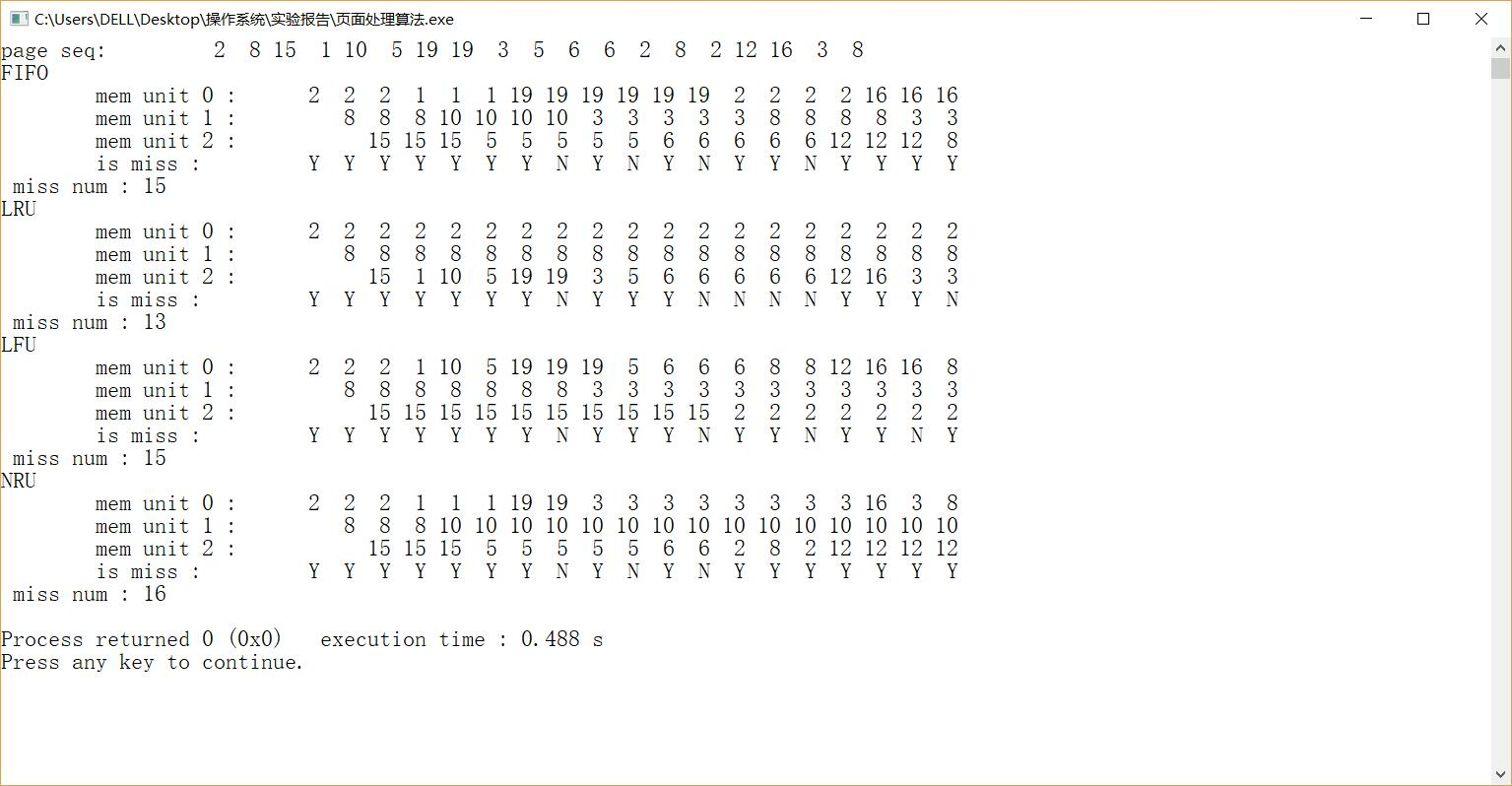六、实验总结：
通过编程模拟四个页面淘汰算法的操作，实际了解了四个算法的淘汰方法以及核心思路，更了解了他们之间的关系和不同点。通过输入不同的数据比较四种方法的效率，确定的他们适用的情况。


展开全文LRU算法
• } } } LFU #include "LFU.h" #include #include #include #include //LFU（Least Frequently Used ，最近最少使用算法 void LFU::execute() {//逻辑也基本一致，区别在于页面的换入换出函数那里 readData("./input/...
项目的结构job工作类头文件#ifndef JOB_H
#define JOB_H

#include <iostream>
#include <vector>
using namespace std;

class Job
{
public:
Job();

/*
父类的一些函数，子类如果想要在自己的构造函数中初始化某些内容，
可以声明来使用：如子类FIFO:
FIFO:FIFO():Job() */
void writeData(string dest);
void show();

bool isContain(int page);

//每个子类中都有不同的execute和查页面的实现，所以要在父类中定义成虚函数，子类继承实现
virtual void execute() = 0;
virtual int SolveJobPage(int curLocation) = 0;

protected:
//定义数据结构
int miss;//记录缺页次数
int memerySize;//可用的物理块个数
int curMemorySize; //当前调入主存中的页面个数
vector<int> records;//作业页面集
vector<int> memory;//内存页面集

vector<vector<int>> memroyRecords;
vector<bool> contain;//缺页标识

private:
};

#endif // JOB_H


四个子类的头文件基本类似，继承job的头文件算法的具体实现和job类的具体实现
job
#include <iostream>
#include <fstream>
#include <cstdlib>
#include <ostream>
#include "Job.h"

Job::Job()
{
//ctor构造类型初始化
memerySize = 3;//定义物理块个数3个
curMemorySize = 0;//当前调入主存的页面个数初始化为0
miss = 0 ;//缺页次数初始化0
memory = vector<int>(3,0);//内存页面集初始化

}

/*Job::Job(){

memory = vector<int>(3,0);
memerySize = 3;
curMemorySize = 0;
miss = 0;
}
*/
{
ifstream infile;//文件以输入方式打开，文件数据输入到内存
infile.open(source.c_str());/*string类对象的成员函数c_str()这里c_str() 以 char* 形式传回 string 内含字符串
当需要打开一个由用户自己输入文件名的文件时，可以这样写：ifstream in(st.c_str());。
其中st是string类型，存放的即为用户输入的文件名。*/
string record;
while(infile >> record){
records.push_back(atoi(record.c_str()));
//push_back() 在Vector最后添加一个元素
//atoi是cstring字符处理函数，把数字字符串转换成int输出
//atoi()的参数是char,对于一个字符串str须调用 c_str()把这个string转换成 const char*类型的
}
}

void Job::writeData(string destination) //实现Job类里的写文件函数
{
ofstream outfile;
outfile.open(destination);
//文件以输出方式打开(内存数据输出到文件)
for(int i=0; i<records.size(); i++)//打印读出来的页面序列
{
if(i == 0){
outfile << "页面序列为" << '\t' << records[i] << "\t";// \t后移一个制表位置
}else{
outfile << records[i] << "\t";
}
}
outfile<<endl;

for(int i=0; i < memroyRecords.size(); i++)//打印每一个物理块随主存调入页面的改变序列
{
for(int j=0; j<memroyRecords.size();j++)
{
if(j == 0){
outfile<<"第"<< i+1<<"物理块"<< '\t' << memroyRecords[j][i] <<"\t";
}else{
outfile << memroyRecords[j][i]<<"\t";
}
}
outfile<<endl;

}
for(int i=0; i<records.size(); i++)//打印缺页情况
{
if(i == 0){
outfile << "是否缺页" << '\t';
}
if(!contain[i]){
outfile <<" \t";
}else{
outfile << "缺\t";
}
}
outfile<<endl;
outfile<<endl;
outfile << "缺页率：" << miss << "\\" << records.size()<<endl; // \\转义的除号

}

void Job::show()
{
for(int i=0; i<records.size(); i++)//打印读出来的页面序列
{
if(i == 0){
cout << "页面序列为" << '\t' << records[i] << "\t";// \t后移一个制表位置
}else{
cout << records[i] << "\t";
}
}
cout<<endl;

for(int i=0; i < memroyRecords.size(); i++)//打印每一个物理块随主存调入页面的改变序列
{
for(int j=0; j<memroyRecords.size();j++)
{
if(j == 0){
cout<<"第"<< i+1<<"物理块"<< '\t' << memroyRecords[j][i] <<"\t";
}else{
cout << memroyRecords[j][i]<<"\t";
}
}
cout<<endl;

}
for(int i=0; i<records.size(); i++)//打印缺页情况
{
if(i == 0){
cout << "是否缺页" << '\t';
}
if(!contain[i]){
cout <<" \t";
}else{
cout << "缺\t";
}
}

cout<<endl;
cout<< "缺页率：" << miss << "\\" << records.size()<<endl; // \\转义的除号
}

bool Job::isContain(int page)//判断命中
{
int flag = false;
//页面号命中物理块号中的序列就是不缺页
for(int i=0; i<memerySize; i++){
if(page == memory[i]){
flag = true;
break;
}
}
return flag;
}


FIFO
#include "FIFO.h"
#include <iostream>
#include <fstream>
#include <ostream>
#include <cstdlib>
#include <algorithm>
#include <cstring>
using namespace std;

void FIFO::execute()//子类实现自己的执行函数
{

//读入数据
bool flag1 =false;//设置一个缺页标志位初始化位缺页
int curPage;//当前页面

for(int i=0; i<records.size(); i++)
{
curPage = records[i];
flag1 =false;

if(!isContain(curPage))//这里用了父类中的布尔判断
{
if (curMemorySize < memerySize)
{
memory[curMemorySize++] = records[i];
flag1 = true;
} else {
int pageIndex =SolveJobPage(i);
memory[pageIndex] = curPage;
flag1 = true;
}

}

contain.push_back(flag1);//加入缺页的标志位
if(flag1){
miss++;
order.push(curPage);
}

memroyRecords.push_back(memory);
}

writeData("./output/FIFO.txt"); //写出数据到文件

}
int FIFO::SolveJobPage(int curLocation){
int tmp = order.front();
order.pop();
for(int i=0; i<memerySize; i++){
if(tmp == memory[i]){
return i;
}
}
}


LFU
#include "LFU.h"

#include <fstream>
#include <ostream>
#include <cstdlib>
#include <cstring>

//LFU（Least Frequently Used ，最近最少使用算法
void LFU::execute()
{//逻辑也基本一致，区别在于页面的换入换出函数那里
//读入数据
bool flag2=false;//设置一个缺页标志位初始化位缺页
int curPage;//当前页面

for(int i=0; i<records.size(); i++)
{
curPage = records[i];
flag2 =false;

if(!isContain(curPage))//
{
if (curMemorySize < memerySize)
{
memory[curMemorySize++] = records[i];
flag2 = true;
} else {
int pageIndex =SolveJobPage(i);
memory[pageIndex] = curPage;
flag2 = true;
}

}

contain.push_back(flag2);//加入缺页的标志
if(flag2){
miss++;
}

memroyRecords.push_back(memory);
}

writeData("./output/LFU.txt"); //写出数据到文件
}

int LFU::SolveJobPage(int curLocation)
{
vector<int> num(memerySize,0);//其实就是3个整型元素的向量，每一个的初始值定义为0
int max = 0;
int index = 0;
for(int i=0; i<memerySize; i++)
{
for(int j=0; j<curLocation; j++){
if(memory[i] == records[j]){
num[i]++;
}
}
}

for(int i=0; i < memerySize; i++){
if(max > num[i]){
index = i;
max = num[i];
}
}
return index;
}


LRU
#include "LRU.h"

#include <fstream>
#include <ostream>
#include <cstdlib>
#include <cstring>

//LRU（The Least Recently Used，最近最久未使用算法
void LRU::execute()
{//这个函数和前面的逻辑也基本一致，区别在于页面的换入换出函数那里
//读入数据
bool flag2=false;//设置一个缺页标志位初始化位缺页
int curPage;//当前页面

for(int i=0; i<records.size(); i++)
{
curPage = records[i];
flag2 =false;

if(!isContain(curPage))//
{
if (curMemorySize < memerySize)
{
memory[curMemorySize++] = records[i];
flag2 = true;
} else {
int pageIndex =SolveJobPage(i);
memory[pageIndex] = curPage;
flag2 = true;
}

}

contain.push_back(flag2);//加入缺页的标志
if(flag2){
miss++;
}

memroyRecords.push_back(memory);
}

writeData("./output/LRU.txt"); //写出数据到文件
}

int LRU::SolveJobPage(int curLocation)
{
vector<int> recordIndex(memerySize,-1);//其实就是3个整型元素的向量，每一个的初始值定义为-1
int max = 1000;
int index = 0;
for(int i=0; i<memerySize; i++)
{
for(int j=0; j<curLocation; j++){
if(memory[i] == records[j]){
recordIndex[i] = j;
}
}
}

for(int i=0; i < memerySize; i++){
if(max > recordIndex[i]){
index = i;
max = recordIndex[i];
}
}
return index;
}

OPT
#include "OPT.h"

#include <fstream>
#include <ostream>
#include <cstdlib>
#include <cstring>

void OPT::execute()//子类实现自己的执行函数
//这个函数和前面FIFO的逻辑基本一致，区别在于下面的处理工作页面的换入换出
{

//读入数据
bool flag2=false;//设置一个缺页标志位初始化位缺页
int curPage;//当前页面

for(int i=0; i<records.size(); i++)
{
curPage = records[i];
flag2 =false;

if(!isContain(curPage))//
{
if (curMemorySize < memerySize)
{
memory[curMemorySize++] = records[i];
flag2 = true;
} else {
int pageIndex =SolveJobPage(i);
memory[pageIndex] = curPage;
flag2 = true;
}

}

contain.push_back(flag2);//加入缺页的标志
if(flag2){
miss++;
}

memroyRecords.push_back(memory);
}

writeData("./output/OPT.txt"); //写出数据到文件
}
//子类自己的页面换入换出处理
int OPT::SolveJobPage(int curLocation){
vector<int> recordIndex(memerySize,1000);//其实就是3个整型元素的向量，每一个的初始值定义为1000
for(int i=0; i<memerySize; i++){

for(int j = records.size()-1; j>curLocation; j--){
if(records[j] == memory[i] ){
recordIndex[i] = j;      // 记录页面下一次出现的位置，，
//其实就是先知倒着看页面出现经过多少次后又被访问了，用来判断哪一个未来最长时间不被使用把他换掉
}
}
}

int max = -1;
int index = 0;
for(int i=0; i<memerySize; i++){
if(max < recordIndex[i]){
index = i;
max = recordIndex[i];
}
}
return index;
}

main方法
#include <iostream>
#include "OPT.h"
#include "FIFO.h"
#include "LFU.h"
#include "LRU.h"
using namespace std;

int main()
{

FIFO fifo;
fifo.execute();
cout<<"FIFO"<<endl;
fifo.show();

LRU lru;
lru.execute();
cout<<"LRU"<<endl;
lru.show();

LFU lfu;
lfu.execute();
cout<<"LFU"<<endl;
lfu.show();

OPT opt;
opt.execute();
cout<<"OPT"<<endl;
opt.show();

return 0;
}

运行结果输入和输出的文件示例展开全文数据结构 c++
• 操作系统课程设计作品，请求分页存储管理的页面置换算法模拟。共四种：FIFO\LRU\LFU\OPT 。在VC环境下运行完全成功。 存储管理的主要功能之一是合理地分配空间。请求页式管理是一种常用的虚拟存储管理技术。本实验...
• Java，操作系统课程设计，模拟页面置换，支持opt,lru,lfu,fifo,
• 常用的页面置换算法有以下几种： 最佳置换算法OPT 先进先出算法FIFO 近期最久未用过算法LRU CLOCK置换算法NRU 页面缓冲算法PBA 近期最少使用算法LFU OPT： 根据未来使用情况将未来的近期里不用的页替换出去...
目标：把未来不再使用的或短期内较少使用的页面调出，通常只能在局部性原理指导下依据过去的统计数据进行预测。
常用的页面置换算法有以下几种：
最佳置换算法OPT
先进先出算法FIFO
近期最久未用过算法LRU
CLOCK置换算法NRU
页面缓冲算法PBA
近期最少使用算法LFU
OPT：
根据未来使用情况将未来的近期里不用的页替换出去。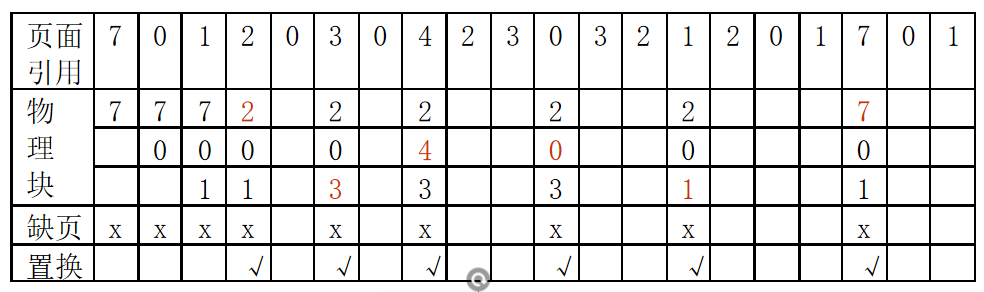举例：发生了6次页面置换，9次缺页中断，总访问次数20次，缺页率9/20＝45％ 。
以上述数据为例，缺页即为物理块中没有下一个页面需要的元素，置换即为物理块中有所需元素。
FIFO
选择内存中驻留最久的页进行淘汰。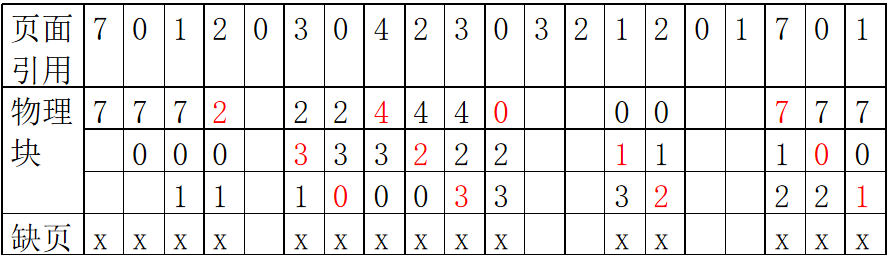以上述数据为例：
发生了12次页面置换，15次缺页中断，总访问次数20次，缺页率15/20＝75％ 。
LRU
近期最久未访问过的页，进行置换。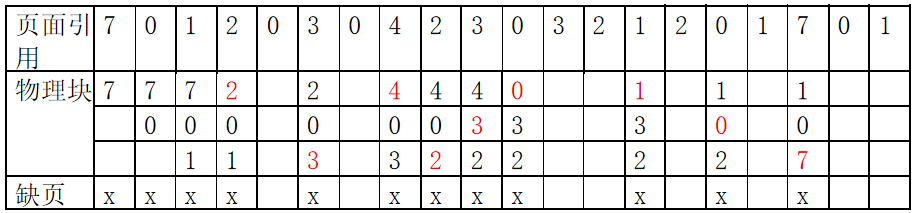最久未访问过的页表示的，701进入，2进入，满，选择最久未访问过的，在2之前，7两次未访问，0一次，1无，故淘汰7，继续，0进入，不缺页，3进入时，1比2 多经过一轮，故1淘汰置换，以此类推。
发生了9次页面置换，12次缺页中断，总访问次数20次，缺页率12/20＝60％ 。
Clock
主要整理一下Clock算法，首先是基础的Clock置换算法，，为每页设置一位访问位，再将内存中的所有页面都通过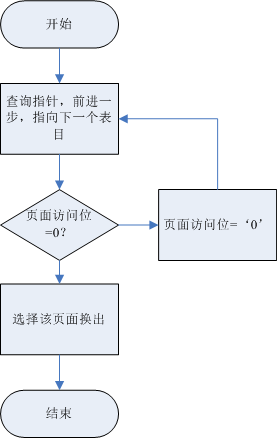这里要注意一点的是，Clock算法只有一个指针，只能用它表示该页是否已经使用过，简单来讲就是，如果是0，就换出，如果是1就置为0，可以先不换出，然后第二轮根据FIFO 算法，即驻留时间最久的换出。
注：如果检查到最后一个页面还是1，再返回队首第一个重新检查。
举例子：2-3-2   进入三个物理块，箭头表示指针
首先是 2⬅
然后是2，3⬅
进入2的时候此时物理块中含有2故不需要页面置换，所以指针不动，仍在3上，并不是在第三个物理块上。
改进Clock
与之前基础的clock算法不同，改进的clock增加了一个注重点，就是置换代价，打个比方，如果该页被修改过，则有置换的必要，如果没有被修改，就没有必要置换，就是一个代价问题。
简单说就是之前只看访问0或者1，现在不仅看访问而且看修改0或者1；
最优就是访问0 and 修改 0，其次是01，10，11；以此来置换


展开全文• 页面置换算法的例题详解必知**最佳(Optimal)置换算法****先进先出(FIFO)页面置换算法****最久未使用(LRU)页面置换算****最少使用(LFU)置换算法**例题一：**题目：****解题：**解题思路： 必知 最佳(Optimal)置换算法...
• 实现了六种页面置换算法：最优算法（OPT）、先来先服务（FIFO)、最近最久未使用（LRU）、时钟算法（CLOCK）、近期最少使用（LFU）、最近最不经常使用（NRU）
• 缓存算法(页面置换算法)-FIFO、LFU、LRU在前一篇文章中通过leetcode的一道题目了解了LRU算法的具体设计思路，下面继续来探讨一下另外两种常见的Cache算法：FIFO、LFU1.FIFO算法FIFO(First in First out)，先进先出。...
• 前言 学习笔记 void IntoPage(int m);...//最佳置换算法（OPT） void FIFO(int num,int Msize);//先进现出置换算法（FIFO） void LRU(int num,int Msize);//最近最久未使用置换算法（LRU） void LFU(int num,int Msize
• 其实在操作系统的设计理念中很多地方都利用到了先进先出的思想，比如作业调度(先来先服务)，为什么这个原则在很多地方都会用到呢？因为这个原则简单、且符合人们的惯性思维，具备公平性，并且实现起来简单，直接使用...
• 主要的页面置换算法有:OPT、LRU、LFU、NUR以及FIFO。FIFO:先进先出算法。OPT: 最佳置换算法。LRU:最近最久未使用算法。LFU:最近最少使用算法。NUR: 最近未使用算法。以上五种算法的具体实现均在体现在以下程序中#...
• 文章目录一、最佳置换算法（OPT）二、先进先出页面置换算法（FIFO）三、最近最久未使用置换算法（LRU）四、最少使用置换算法（LFU）五、Clock置换算法（最近未使用算法 NRU）六、页面缓冲算法（PBA）七、访问内存的...虚拟存储器
• 页面置换算法分为两类 1、局部页面置换算法 最优页面置换算法（OPT、optimal） 先进先出算法（FIFO） 最近最久未使用算法（LRU,Least Recently Used） 时钟页面置换算法（Clock） 最不常用算法（LFU，Least ...
• 操作系统的内存管理中，有一类很重要的算法就是内存页面置换算法（包括FIFO，LRU,LFU等几种常见页面置换算法）。事实上，Cache算法和内存页面置换算法的核心思想是一样的：都是在给定一个限定大小的空间的前提下，...LRU
• 操作系统课程设计报告-页面置换算法模拟系统，模拟了进先出的算法（FIFO），最佳淘汰算法（OPT），最近最久未使用算法（LRU），最少访问页面算法（LFU），并含有DOS界面的菜单选择模块
• 缓存算法（页面置换算法）-FIFO. LFU. LRU 在前一篇文章中通过leetcode的一道题目了解了LRU算法的具体设计思路，下面继续来探讨一下另外两种常见的Cache算法：FIFO. LFU 1.FIFO算法 FIFO（First in First out...
• 缓存算法（页面置换算法）-FIFO、LFU、LRU 　在前一篇文章中通过leetcode的一道题目了解了LRU算法的具体设计思路，下面继续来探讨一下另外两种常见的Cache算法：FIFO、LFU 1.FIFO算法 　FIFO（First in First out...
• 下面介绍在虚拟存储管理中有哪些页面置换算法。 1.总览 局部页面置换算法 最优页面置换算法（OPT，optimal） 先进先出算法（FIFO） 最近最久未使用算法（LRU，Least Recently Used） 时钟页面置换算法...